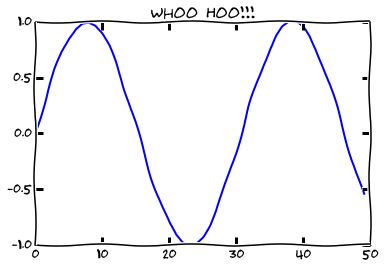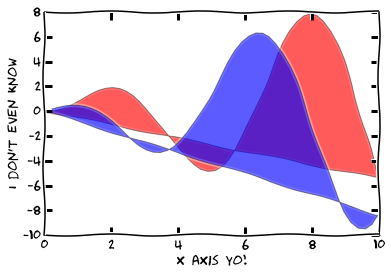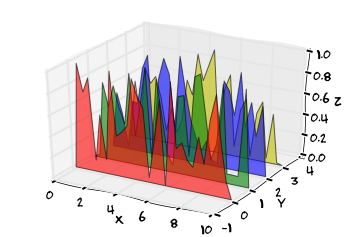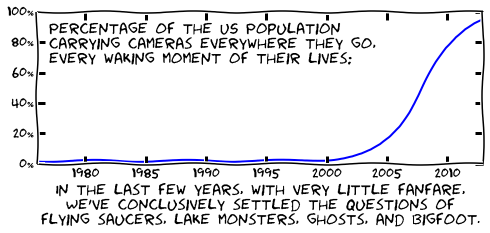# XKCD Plots have Landed in Matplotlib!

One of the most consistently popular posts on this blog has been my XKCDify post, where I followed in the footsteps of others to write a little hack for xkcd-style plotting in matplotlib. In it, I mentioned the Sketch Path Filter pull request that would eventually supersede my ugly little hack.

Well, "eventually" has finally come. Observe:

In :
%pylab inline

Welcome to pylab, a matplotlib-based Python environment [backend: module://IPython.kernel.zmq.pylab.backend_inline].

In :
plt.xkcd()  # Yes...
plt.plot(sin(linspace(0, 10)))
plt.title('Whoo Hoo!!!')

Out:
<matplotlib.text.Text at 0x2fade10>The plt.xkcd() function enables some rcParam settings which can automatically convert any matplotlib plot into XKCD style. You can peruse the matplotlib xkcd gallery here for inspiration, or read on where I'll show off some of my favorite of the possibilities.

By the way, this new functionality requires matplotlib version 1.3, which can currently be downloaded and installed from github. Also, if you want to have the font match above, be sure to download and install the Humor Sans font on your system. For matplotlib to recognize it, you may have to remove the font cache, found on your system at

\$HOME/.matplotlib/fontList.cache

Even simple plots can be made much more interesting:

In :
x = np.linspace(0, 10)
y1 = x * np.sin(x)
y2 = x * np.cos(x)

plt.fill(x, y1, 'red', alpha=0.4)
plt.fill(x, y2, 'blue', alpha=0.4)
plt.xlabel('x axis yo!')
plt.ylabel("I don't even know")

Out:
<matplotlib.text.Text at 0x31e3190>3D plots work as well:

In :
from mpl_toolkits.mplot3d import Axes3D
from matplotlib.collections import PolyCollection
import matplotlib.pyplot as plt
import numpy as np

fig = plt.figure()
ax = fig.gca(projection='3d')

x = np.linspace(0, 10, 30)
colors = ['r', 'g', 'b', 'y']
y = np.random.random((len(colors), len(x)))
y[:, 0] = y[:, -1] = 0

edges = [list(zip(x, yi)) for yi in y]

poly = PolyCollection(edges, facecolors=colors, alpha=0.6)

ax.set_xlabel('X')
ax.set_xlim3d(0, 10)
ax.set_ylabel('Y')
ax.set_ylim3d(-1, 4)
ax.set_zlabel('Z')
ax.set_zlim3d(0, 1)

/home/vanderplas/PythonEnv/pydev/local/lib/python2.7/site-packages/matplotlib-1.3.x-py2.7-linux-x86_64.egg/matplotlib/axis.py:695: MatplotlibDeprecationWarning: This function has been made private and movedto _set_scale. This wrapper function will be removed in 1.4
"removed in 1.4", mplDeprecation)

Out:
(0, 1)We can use the new package to quickly reproduce a recent XKCD comic:

In :
fig = plt.figure(figsize=(8, 6))

years = np.linspace(1975, 2013)
pct = 2 + 98. / (1 + np.exp(0.6 * (2008 - years)))
ax.plot(years, pct)

ax.set_xlim(1976, 2013)
ax.set_ylim(0, 100)
ax.yaxis.set_major_formatter(plt.FormatStrFormatter('%i%%'))

ax.text(1977, 67,
("Percentage of the US Population\n"
"carrying cameras everywhere they go,\n"
"every waking moment of their lives:"),
size=16)

ax.set_xlabel(("In the last few years, with very little fanfare,\n"
"We've conclusively settled the questions of\n"
"flying saucers, lake monsters, ghosts, and bigfoot."),
size=16)

Out:
<matplotlib.text.Text at 0x35adc90>And as my favorite example, we can recreate any of the animations I've shown in this blog, making them xkcd-style with a simple plt.xkcd() annotation. Below you can see the double-pendulum animation from this post, rendered using the XKCD sketch path:

In :
from IPython.display import HTML